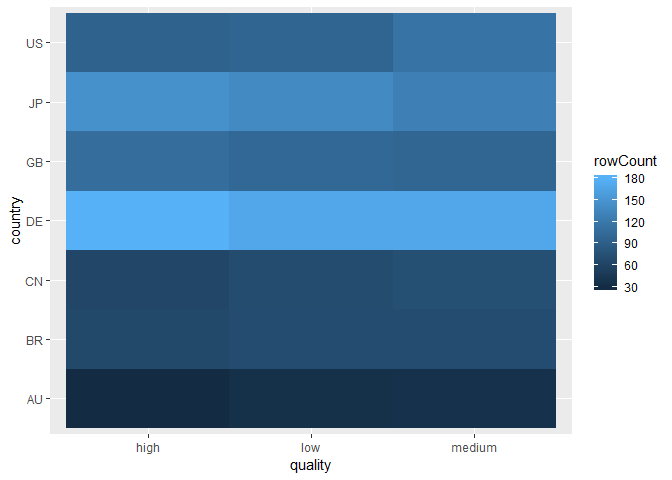Descriptive analysis (sometimes referred to as Exploratory analysis) is a crucial part in any kind of analysis. The goal is to “get to know your dataset” - to find outliers, irregularities and identify what transformations to do for the further stages (e.g. for modelling). Exploratory phase is largely an iterative process, where you develop understanding of the data by

• collecting questions about the data
• answering them by transforming the data, summarizing and plotting it,
• which leads to new questions :)

## Prerequisites (recap)

The power of R comes from the vast universe of packages. Every time you need a new package you install it:

install.packages("ggplot2")
install.packages("dplyr")

library("ggplot2")
library("dplyr")

Now we load a dataset into R. Today we are analyzing data (artificial) of total sales on a weekly basis for two particular products in different stores. There are many ways things can be done in R. For example, you can read in dataset using basic functionality:

There are a planty of ways to load data from .csv files. The one that we will use:

df <- read.csv(file.choose()) # lab2_Data.csv

Let’s print some rows and look at the data that we loaded:

head(df,3)
##   storeNum Year Week p1sales p2sales p1price p2price p1prom p2prom country
## 1      101    1    1     127     106    2.29    2.29      0      0      US
## 2      101    1    2     137     105    2.49    2.49      0      0      US
## 3      101    1    3     156      97    2.99    2.99      1      0      US

Let us understand the dataset.

View(df)

We can use another functions to study our data:

str(df)
## 'data.frame':    2080 obs. of  10 variables:
##  $storeNum: int 101 101 101 101 101 101 101 101 101 101 ... ##$ Year    : int  1 1 1 1 1 1 1 1 1 1 ...
##  $Week : int 1 2 3 4 5 6 7 8 9 10 ... ##$ p1sales : int  127 137 156 117 138 115 116 106 116 145 ...
##  $p2sales : int 106 105 97 106 100 127 90 126 94 91 ... ##$ p1price : num  2.29 2.49 2.99 2.99 2.49 2.79 2.99 2.99 2.29 2.49 ...
##  $p2price : num 2.29 2.49 2.99 3.19 2.59 2.49 3.19 2.29 2.29 2.99 ... ##$ p1prom  : int  0 0 1 0 0 0 0 0 0 0 ...
##  $p2prom : int 0 0 0 0 1 0 0 0 0 0 ... ##$ country : Factor w/ 7 levels "AU","BR","CN",..: 7 7 7 7 7 7 7 7 7 7 ...
dim(df)
##  2080   10

Now find all the countries where stores are located.

## Removing NA values

Let’s check for a NA:

rows <- which(is.na(df), arr.ind=TRUE)[,1]
df[rows,]
##    storeNum Year Week p1sales p2sales p1price p2price p1prom p2prom
## 12      101    1   12      NA      73    2.49    3.19      0      0
## 33      101    1   33     120      77    2.79    2.99      0      0
##    country
## 12      US
## 33    <NA>

We can use next function to remove rows with NA:

df <- na.omit(df)

rows <- which(is.na(df), arr.ind=TRUE)[,1]
df[rows,]
##   storeNum Year     Week     p1sales  p2sales  p1price  p2price
##   p1prom   p2prom   country
## <0 rows> (or 0-length row.names)

## Descriptive statistics

Summary statistics is displayed by:

summary(df)
##     storeNum          Year          Week          p1sales
##  Min.   :101.0   Min.   :1.0   Min.   : 1.00   Min.   : 73
##  1st Qu.:106.0   1st Qu.:1.0   1st Qu.:14.00   1st Qu.:113
##  Median :111.0   Median :2.0   Median :26.50   Median :129
##  Mean   :110.5   Mean   :1.5   Mean   :26.50   Mean   :133
##  3rd Qu.:115.8   3rd Qu.:2.0   3rd Qu.:39.75   3rd Qu.:150
##  Max.   :120.0   Max.   :2.0   Max.   :52.00   Max.   :263
##
##     p2sales         p1price         p2price          p1prom
##  Min.   : 51.0   Min.   :2.190   Min.   :2.290   Min.   :0.0000
##  1st Qu.: 84.0   1st Qu.:2.290   1st Qu.:2.490   1st Qu.:0.0000
##  Median : 96.0   Median :2.490   Median :2.590   Median :0.0000
##  Mean   :100.2   Mean   :2.544   Mean   :2.699   Mean   :0.1001
##  3rd Qu.:113.0   3rd Qu.:2.790   3rd Qu.:2.990   3rd Qu.:0.0000
##  Max.   :225.0   Max.   :2.990   Max.   :3.190   Max.   :1.0000
##
##      p2prom       country
##  Min.   :0.0000   AU:104
##  1st Qu.:0.0000   BR:208
##  Median :0.0000   CN:208
##  Mean   :0.1386   DE:520
##  3rd Qu.:0.0000   GB:312
##  Max.   :1.0000   JP:416
##                   US:310

We see that country is a factor and represents country where store is located. There are 7 different countries and we can see amount of observations with each country after “:”.

We can study columns using next functions:

unique(df$country)# displays all unique values ##  US DE GB BR JP AU CN ## Levels: AU BR CN DE GB JP US length(unique(df$storeNum)) # number of unique countries in our dataset
##  20

## Discrete variables

Another useful inspection for discrete features (variables) is using frequency counts. This can be achieved by table function:

table(df$p1prom) ## ## 0 1 ## 1870 208 table(promotion_p1=df$p1prom, promotion_p2=df$p2prom) # two-way frequency table ## promotion_p2 ## promotion_p1 0 1 ## 0 1614 256 ## 1 176 32 The next question we might ask is how much varies price of the first and second product: summary(df$p1price)
##    Min. 1st Qu.  Median    Mean 3rd Qu.    Max.
##   2.190   2.290   2.490   2.544   2.790   2.990
summary(df$p2price) ## Min. 1st Qu. Median Mean 3rd Qu. Max. ## 2.290 2.490 2.590 2.699 2.990 3.190 We also would like to know what are the prices when the product’s price is reduced and is not. Price is a continuous variable. However, we already checked that it does not vary much. We can display frequencies using table function: price_table <- table(promotion=df$p1prom, price=df$p1price) # how many times each product was promoted at each price level price_table ## price ## promotion 2.19 2.29 2.49 2.79 2.99 ## 0 354 398 380 395 343 ## 1 41 46 42 47 32 But let’s see the percentages and margins instead of the counts. This way, we can compare it to the second product. # promotions are in rows, prices are in columns # summing over rows margin.table(price_table, 1) ## promotion ## 0 1 ## 1870 208 # summing over columns margin.table(price_table, 2) ## price ## 2.19 2.29 2.49 2.79 2.99 ## 395 444 422 442 375 prop.table(price_table) # cell % ## price ## promotion 2.19 2.29 2.49 2.79 2.99 ## 0 0.17035611 0.19153032 0.18286814 0.19008662 0.16506256 ## 1 0.01973051 0.02213667 0.02021174 0.02261790 0.01539942 prop.table(price_table, 1) # row percentages ## price ## promotion 2.19 2.29 2.49 2.79 2.99 ## 0 0.1893048 0.2128342 0.2032086 0.2112299 0.1834225 ## 1 0.1971154 0.2211538 0.2019231 0.2259615 0.1538462 prop.table(price_table, 2) # column percentages ## price ## promotion 2.19 2.29 2.49 2.79 2.99 ## 0 0.89620253 0.89639640 0.90047393 0.89366516 0.91466667 ## 1 0.10379747 0.10360360 0.09952607 0.10633484 0.08533333 Find now the percentages of discounted products for different price levels for product 2. ## Continuous variables For continuous variables, where data varies a lot, table is not feasible nor meaningful. Try for example running table(dt$p1sales). The following table summarizes the functions of descriptive statistics that we discussed during the lecture.

• min(x) - Minimum value
• max(x) - Maximum value
• mean(x) - Mean
• median(x) - Median
• var(x) - Variance
• sd(x) - Standard deviation
• quantile(x, probs=c(0.25, 0.5, 0.75)) - Percentiles/Quartiles
• cor(x, y) Correlation function

Observe statistics for sales of product1. Is there anything interesting?

## Data Transformations

Often exploring data involves a narrower approach. For example, instead of average sales of the first product we want to calculate average sales for each country, or for each shop. Or we want to filter the data and take a look at average sales when the product was promoted. If you have experience with other programming languages, your first approach for such analysis would be to run for loops. However, the power of R comes from the vectorized approach.

Vectorization is the process of rewriting a loop so that instead of processing a single element of an array N times, it processes (for example) 4 elements of the array simultaneously N/4 times.

A bit too technical, but let’s see what it means on practice. Let’s first try to calculate average sales per country using classical approach:

countries = unique(df$country) # choose unique countries in our dataset for(cn in countries){ # create a loop to pick a country from our country vector country_df <- filter(df, country==cn) # filter the data, so that only data for this country is chosen country_mean <- mean(country_df$p1sales) # calculate the mean for this country
print(c(cn, country_mean)) # print the result one-by-one
}
##  "US"               "133.703225806452"
##  "DE"               "132.453846153846"
##  "GB"               "131.365384615385"
##  "BR"               "133.826923076923"
##  "JP"               "133.127403846154"
##  "AU"               "139.846153846154"
##  "CN"               "131.639423076923"

Now the vectorized approach would be:

df %>%
group_by(country) %>%
summarise(sales_mean = mean(p1sales))
## # A tibble: 7 x 2
##   country sales_mean
##   <fct>        <dbl>
## 1 AU            140.
## 2 BR            134.
## 3 CN            132.
## 4 DE            132.
## 5 GB            131.
## 6 JP            133.
## 7 US            134.

Let’s measure the time taken for each of two approaches by writing Sys.time around our functions (the first is ‘for loop’ and the second is vectorized approach):

start <- Sys.time()
countries = unique(df$country) # choose unique countries in our dataset for(cn in countries){ # create a loop to pick a country from our country vector country_df <- filter(df, country==cn) # filter the data, so that only data for this country is chosen country_mean <- mean(country_df$p1sales) # calculate the mean for this country
print(c(cn, country_mean)) # print the result one-by-one
}
##  "US"               "133.703225806452"
##  "DE"               "132.453846153846"
##  "GB"               "131.365384615385"
##  "BR"               "133.826923076923"
##  "JP"               "133.127403846154"
##  "AU"               "139.846153846154"
##  "CN"               "131.639423076923"
Sys.time() - start
## Time difference of 0.00408721 secs
start <- Sys.time()
df %>%
group_by(country) %>%
summarise(sales_mean = mean(p1sales))
## # A tibble: 7 x 2
##   country sales_mean
##   <fct>        <dbl>
## 1 AU            140.
## 2 BR            134.
## 3 CN            132.
## 4 DE            132.
## 5 GB            131.
## 6 JP            133.
## 7 US            134.
Sys.time() - start
## Time difference of 0.2389381 secs

And this is on a very tiny dataset! On bigger sizes the difference becomes much more crucial.

## Visualization

Let’s try to practice how to do charts for our later analysis, as we will do a lot of graphs along the course. It is important to remember what is the purpose of your plots. There are plots with the main goal to communicate the message for the decision-makers or consumers. These plots have to clearly convey the message that was found and investigated earlier. For the exploratory analysis the graphs facilitate the discovery. We have to learn how to detect interesting and unexpected patterns and what questions to ask next.

### Bar chart. Multi-set bar chart

The following chart displays how many transactions per country were made.

ggplot(data = df) +
geom_bar(mapping = aes(x = country))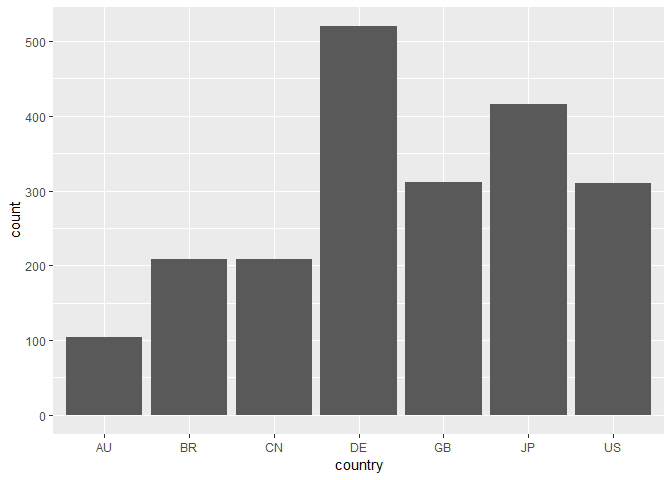Compare it to the results of the table df %>% count(country):

df %>% count(country)
## # A tibble: 7 x 2
##   country     n
##   <fct>   <int>
## 1 AU        104
## 2 BR        208
## 3 CN        208
## 4 DE        520
## 5 GB        312
## 6 JP        416
## 7 US        310

The ggplot2 plots are very flexible. Let’s try to beautify it a bit:

ggplot(data = df) +
geom_bar(mapping = aes(x = country), fill='#f1e8ca',
color='#745151', alpha=0.8) + theme_bw(base_size=28)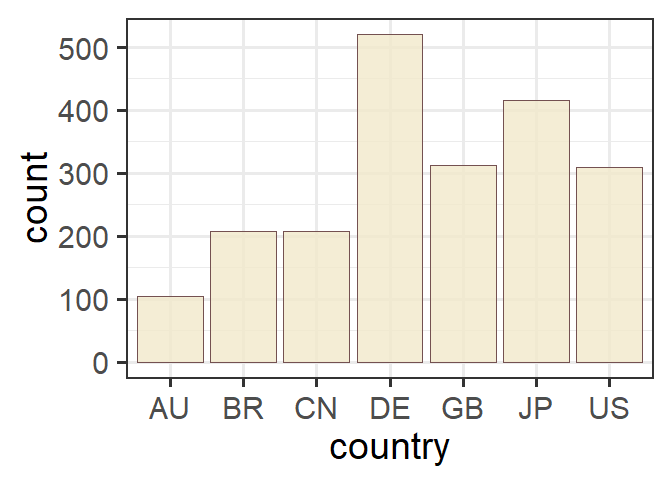Multi-chart bar:

df %>%
mutate(p1prom = as.factor(p1prom)) %>%
ggplot() +
geom_bar(mapping = aes(x = country, fill=p1prom),
position='dodge')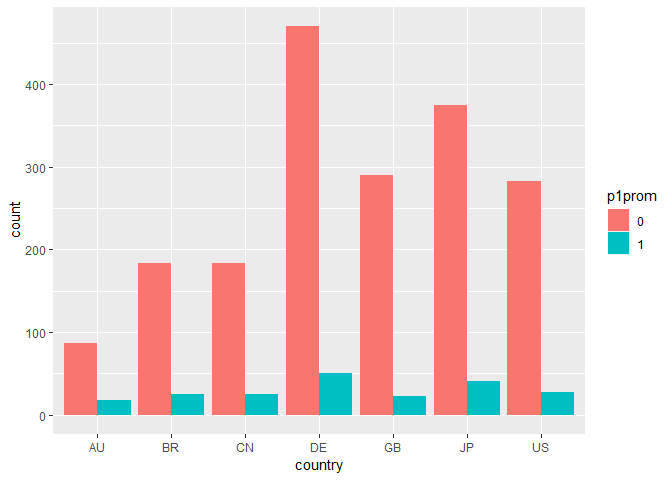How do you interpret this plot? Try to customize it by changing colors (check help for scale_fill_manual())

### Histogram. Density

Histogram shows the distribution of continuous variable. In a histogram the x-axis is partitioned into equal bins. The height of a bar shows how many observations fall into each bin. The results of the histogram heavily depend on the chosen bin size.

ggplot(data = df) +
geom_histogram(mapping = aes(x = p1sales))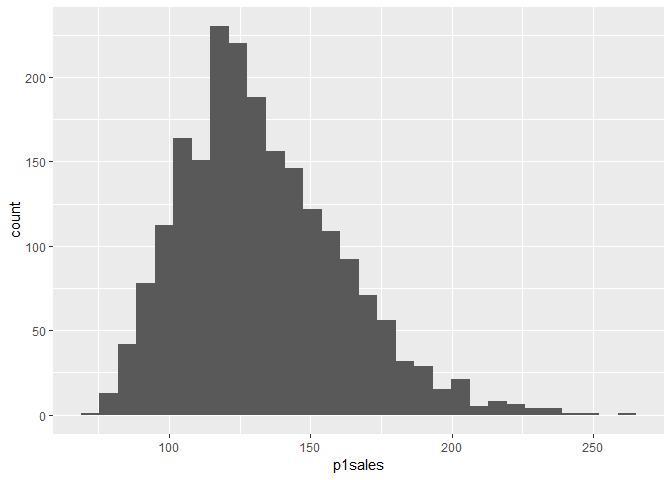ggplot(data = df) +
geom_histogram(mapping = aes(x = p1sales), binwidth=50)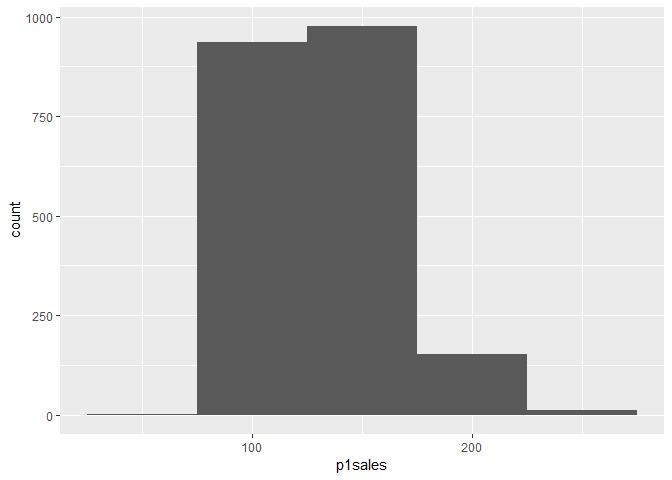ggplot(data = df) +
geom_histogram(mapping = aes(x = p1sales), binwidth=1)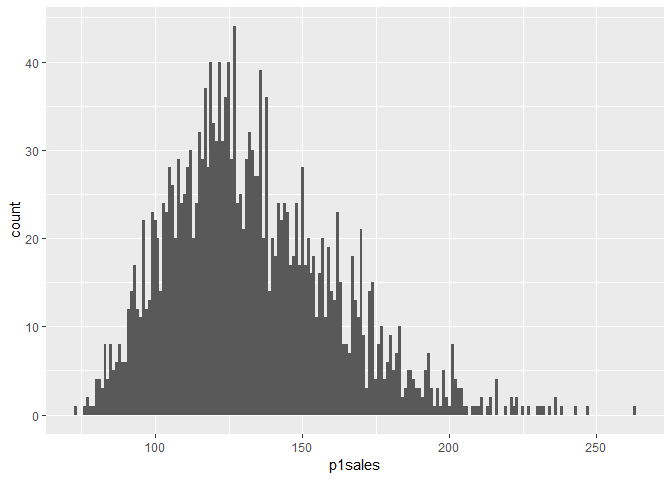Another plot for the distribution is density plot. It is a smoothed histogram that provide a general estimate of the distribution. The area under the distribution is always sums to 1. It helps to estimate general tendency, however it comes at a cost, as y-axis is difficult to interpret.

ggplot(data = df) +
geom_density(mapping = aes(x = p1sales), fill='#f1e8ca')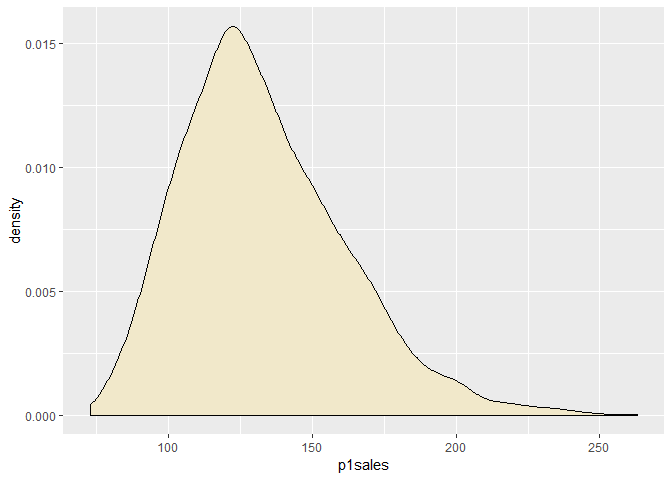It is more helpful to compare several distributions via density plots:

ggplot(data = df) +
geom_density(mapping = aes(x = p1sales), fill='#f1e8ca',
alpha=0.3) +
geom_density(aes(x=p2sales), fill = '#745151', alpha=0.3) + xlab('sales')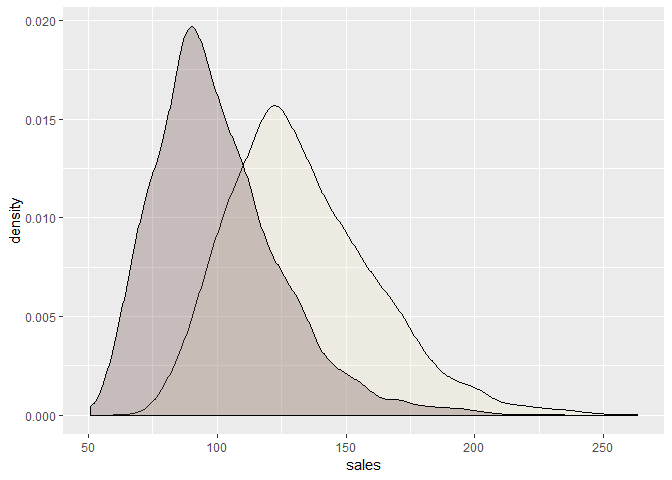### Boxplot

While histogram is a good way to show the distribution of one continuous variable, if we want to compare several distributions, it may become tricky. Densities are good, if there are not too many either. We could overlay several histograms, but if the scale between two histograms vary a lot, it is difficult to analyze. A better approach would be to use boxplots:

ggplot(data = df, mapping = aes(x = as.factor(storeNum), y = p1sales)) + geom_boxplot() + theme_bw() + xlab("storeNum")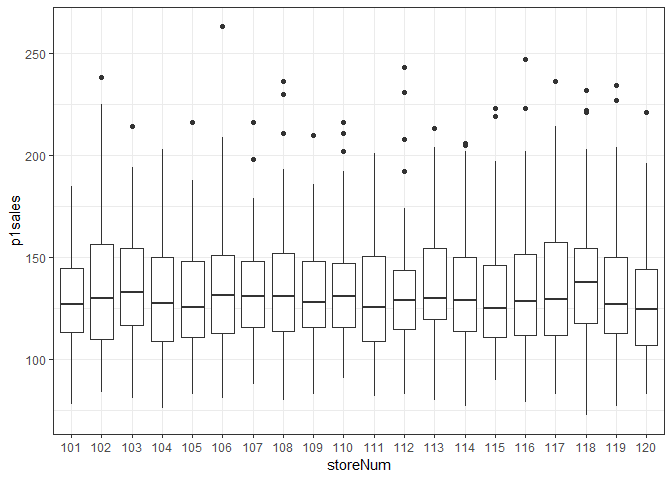Boxplots help to quickly grasp the information about several distributions and compare it.

Try to change geom_boxplot to geom_violin. Use help to check (type ?geom_violin).

### Line chart

Line charts are often the easiest to understand :). Usually, the most common way is to use it for time-series, e.g. we want to observe a behavior over time.

Often line charts are “misused”. Look at the following graph and explain what is wrong with it:

df %>%
group_by(country) %>%
summarise(p1sales=sum(p1sales)) %>%
ggplot(aes(x=country, y=p1sales, group=1)) + geom_line() +
theme_bw()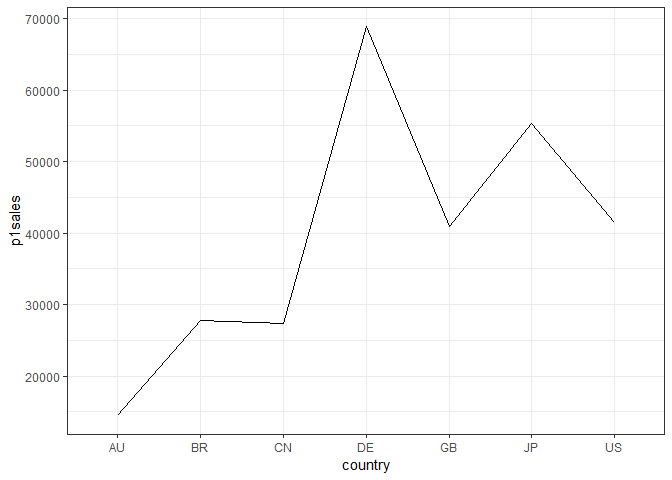### Scatter plot. Correlation

Two continuous variables can be visualized using scatterplot. It shows the relationship between these variables. We will later observe, how such relationship helps in models. Let’s first observe what are the basic patterns we want (or don’t want) to see in the data: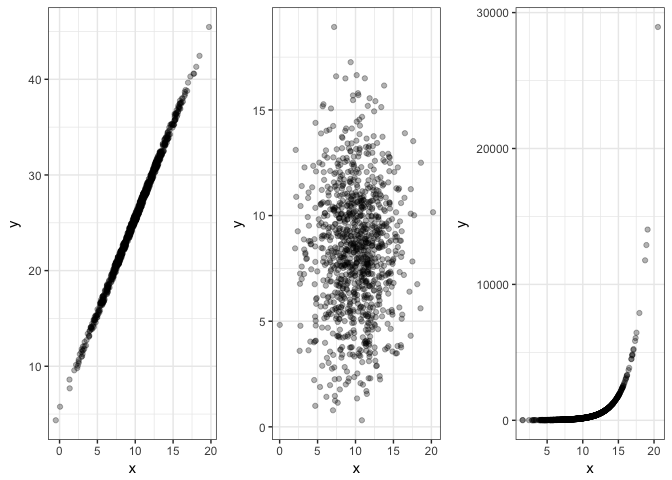The relationship between teo variables can be expressed with the correlation coefficient. However, it only shows whether there is a linear relationship.

Make a scatterplot using product 1 and product 2 sales data. What patterns do you observe?

From graph alone it might be hard to define relationship between variable, so we can use cor() functon to have more determination:

cor(df$p1sales, df$p2sales)
##  -0.5584222

The sign in correlation means in what way first feature affects second (+ for increases; - for decreses).

## Melting/Casting

There is a particular concept of long and wide data formats, as we discussed during the lecture. For example, when we made plots of two density plots for product 1 and product 2, it was not the optimal way to do it. We can tidy our data.

To do so, we will need tidyr package:

install.packages("tidyr")
library("tidyr")
gathered_df <- df %>%
gather(p1sales, p2sales, key = "product", value = "sales")
head(gathered_df)
##   storeNum Year Week p1price p2price p1prom p2prom country product sales
## 1      101    1    1    2.29    2.29      0      0      US p1sales   127
## 2      101    1    2    2.49    2.49      0      0      US p1sales   137
## 3      101    1    3    2.99    2.99      1      0      US p1sales   156
## 4      101    1    4    2.99    3.19      0      0      US p1sales   117
## 5      101    1    5    2.49    2.59      0      1      US p1sales   138
## 6      101    1    6    2.79    2.49      0      0      US p1sales   115

Now we can build the plot with densities in more natural way:

ggplot(data = gathered_df) +
geom_density(mapping = aes(x = sales, fill=product),
alpha=0.3) +
scale_fill_manual(values = c('#f1e8ca', '#745151'))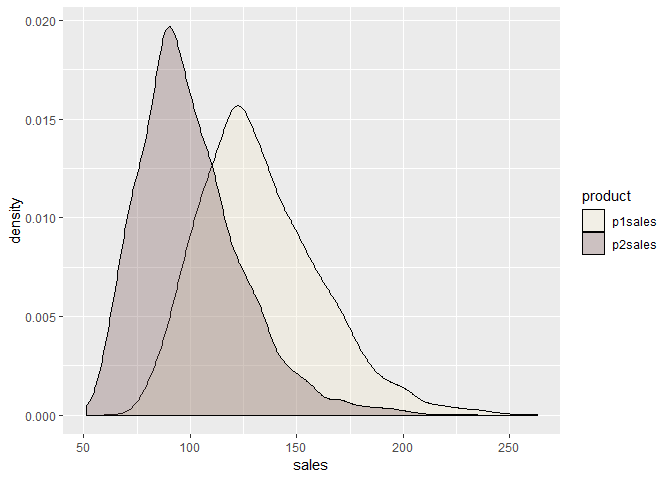## Heatmap

A heat map (or heatmap) is a graphical representation of data where the individual values contained in a matrix are represented as colors. It is really useful to display a general view of numerical data, not to extract specific data point.

In our example, we will add one more column that will display the quality of the shop:

set.seed(13)
df <- cbind(df, quality = sample(as.factor(c("low", "medium", "high")), nrow(df), replace = TRUE))

Now we can build the heatmap:

df %>%
select(quality,country) %>%
group_by(country, quality) %>%
summarize(rowCount= n()) %>%
ggplot() + geom_tile(aes(x = quality, y = country, fill = rowCount))